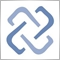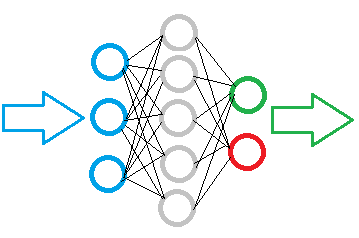# Discussion of article "Neural networks made easy (Part 5): Multithreaded calculations in OpenCL"Moderator
229523

New article Neural networks made easy (Part 5): Multithreaded calculations in OpenCL has been published:

We have earlier discussed some types of neural network implementations. In the considered networks, the same operations are repeated for each neuron. A logical further step is to utilize multithreaded computing capabilities provided by modern technology in an effort to speed up the neural network learning process. One of the possible implementations is described in this article.

After selecting the technology, we need to decide on the process of splitting calculations into threads. Do you remember the fully connected perceptron algorithm during a feed-forward pass? The signal moves sequentially from the input layer to hidden layers and then to the output layer. There is no point in allocating a thread for each layer, as calculations must be performed sequentially. A layer calculation cannot start until the result from the previous layer is received. The calculation of an individual neuron in a layer does not depend on the results of calculation of other neurons in this layer. It means that we can allocate separate threads for each neuron and send all neurons of a layer for parallel computation.Going down to the operations of one neuron, we could consider the possibility of parallelizing the calculation of the products of input values by their weight coefficients. However, further summation of the resulting values and the calculation of the activation function value are combined into a single thread. I decided to implement these operations in a single OpenCL kernel using vector functions.

Author: Dmitriy Gizlyk6951

Thank you for these articles! It's been very interesting following the ideas of this system.

I'm very interested in the LSTM-module, is there a way to use Open-CL for LSTM-Networks?

I've been trying to modify the LSTM-EA to work with Open-CL, but with no success.

Best regards

Pontus